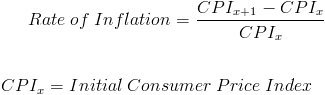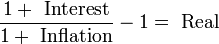Finance and Career# Real Interest vs. Nominal Interest: What’s the Difference?

Start talking about money and interest rates come into the equation.  Get a mortgage, buy a house, or get an auto loan—you will hear about interest rates.  But what you may not know is that the interest rate referenced most of the time is the nominal interest rate, and not the real interest rate.  So what is the difference between real versus nominal interest rates?

The nominal interest rate is also the interest rate that you earn on your money if it is in your savings account, or it is also the interest rate you pay when you borrow money.  So for instance, if you borrow \$10,000 for a loan from a bank and the interest rate charged is 5%, then the nominal interest rate is 5%.

But the problem with nominal interest rates is that it does not take into account the rate of inflation (the rise in price of goods and services).  Inflation is a measure of how much your money is worth when compared to a year in the past.  In essence, the nominal interest rate does take into account the decrease in the value of money.  So the difference between the real interest rate and the nominal interest rate is that the real interest rate takes into account the rate of inflation.

So in our example above you paid 5% to the lender for your \$10,000 loan.  However, if you factor in an inflation rate of 2%, then the real interest rate is 3% (5-2).   So essentially, the interest rate is found by taking the nominal interest rate minus the inflation rate.  To quantify the formula for deriving the real interest rate, here is a more exact equation to figure out the real interest rate:As you can see the equation will tell you to divide 1+nominal interest rate by 1+inflation rate  After dividing, you will subtract 1 from it.  From there, you will get the real interest rate.  The economic formula shown above is just a different expression for deriving the same approximate answer (3%).  However, with that said, the formula shown above will give you a more exact answer for the real interest rate, as opposed to subtracting the inflation rate from the nominal interest rate.

To get the annual inflation rate numbers, you can go to the BLS site.  The annual inflation rate is used to figure out how much the value of money has decreased within the last year.  Alternatively, inflation is also defined as the increase in the price of goods and services.

### Figuring out the Inflation Rate The Hard Way

If you are not satisfied with the inflation numbers from the BLS site and want to derive your own inflation rate, here is the formula.

((CPI1 – CPI 2)/(CPI2)*100)

Here is a graphic for the equation:Essentially, you will take the consumer price index of a year (CPIx+1) minus the consumer price index of the year prior (CPIx).  So if you are trying to find out the rate of inflation to figure out the real interest rate , you would take the annual CPI of 2012 and subtract it from the annual CPI of 2013 (the last full year as of the writing of this article).  You will take that number and divide it by the consumer price index of 2012 (CPIx). Afterwards, you will take that number and multiply it by 100.  The answer you get is the inflation rate, expressed as a percentage.  In order to get the CPI numbers, go to this Bureau of Labor Statistics page.

The example we used above is to figure out the annual inflation rate—the inflation rate within the past year.  This is because we want to figure out the real interest rate.

However, the formula can be used to figure out the rate of inflation over a period of time, say from 1994 to 2012.  All you have to do is take the annual CPI of 1994 and subtract that from the annual CPI of 2012.

## An Example of Calculating the Real  Interest Rate

Let’s just say hypothetically that you are  quoted at 6% for your mortgage.  The 6% is the nominal interest rate.  However, you want to figure out the real interest rate.  When getting the real interest rate number.

So first, what you will do is take the annual CPI of 2012 and subtract it from the CPI of 2013 (the last full year).   The annual CPI of 2013 is 239.207 and the annual CPI of 2012 is 236.648. Once you subtract the 239.207 from 236.648, you get 2.559.  Then you will take 2.559 and divide it by the annual CPI of 2012 (236.648), you get 0.01.  Then you will take 0.01 and multiply by 100, to get 1%.  So if the nominal interest rate is 6% and the inflation rate is 1%, then the real interest rate is 5%.

***

With all this mumbo-jumbo being said about real and nominal interest rate—it really doesn’t matter all that much in our day-to-day lives.  But knowing the difference between the two types of interest rates will allow us to make better financial decisions and build a better framework for how we view money.